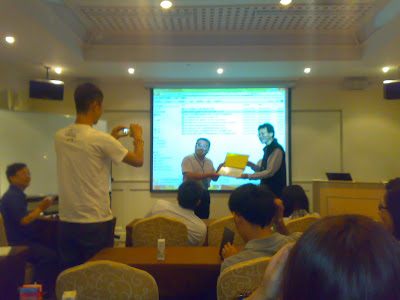## 2010年8月31日 星期二

### 應用 HTML5 及版本控制技術提昇 Web-based 營建資訊管理系統使用效率之研究2010-7-20 參加 2010 營建管理研究會，並發表一篇論文「應用 HTML5 及版本控制技術提昇 Web-based 營建資訊管理系統使用效率之研究」，僥倖獲得評審青睞，拿到一張獎狀。## 2010年8月30日 星期一

### 以 least square method 搜尋非線性方程式的係數值

PDF表文下載

rst全文下載

** 以下純供搜尋引擎使用，生人勿近 **

--------------------------------------------------------------------------------

(1, 4), (4, 5), (1, 2), (4, 5), (6, 9),
(1, 8), (4, 5), (3, 4), (5, 5), (6, 6) 等，

--------------------------------------------------------------------------------

(:math:$(y_1, x_{11}, ..., x_{1k})$, :math:$(y_2, x_{21}, ..., x_{2k})$, ..., :math:$(y_m, x_{m1}, ...x_{mk})$) 下，

.. raw:: latex

\begin{equation}\label{errors define}
f_i(p_1, p_2, ..., p_n) = y_i - E(X_i, p_1, p_2, ..., p_n), i \in 1, ..., m
\end{equation}

\begin{equation}\label{F function}
F(p_1, p_2, ..., p_n) = \sum_{i=0}^{m} (f_i(p_1, p_2, ..., p_n))^2, m \geq n
\end{equation}

.. raw:: latex

\begin{equation}\label{Taylor expansion}
F(P_{k+1}) = F(P_k) + h^{\top} {F}'(P_k) + \frac {1}{2} h^{\top} {F}''(P_k) h + O(||h||^3)
\end{equation}

\begin{equation}
h = P_{k+1} - P_k
\end{equation}

:math:$O(||h||^3)$ 代表後面無窮盡的項目，
:math:$k$ 代表的是第幾次 iteration 。在每次 iteration 中，

Newton's method, Guass-Newton method, The Levenberg-Marquardt method。

The Steepest Descent method
********************************************************************************

Steepest Descent method 將式 :ref:\ref{Taylor expansion} 改寫

.. raw:: latex

\begin{equation}\label{Taylor expansion2}
F(P_{k}+\alpha h) \simeq F(P_k) + \alpha h^{\top} {F}'(P_k), \alpha > 0
\end{equation}

.. raw:: latex

\begin{equation}\label{steepest descent}
\lim_{\alpha \to 0} \frac{F(P) - F(P + \alpha h)}{\alpha \left \| h \right \|}
= - \frac{1}{\alpha \left \| h \right \|} h^{\top} {F}'(P)
= - \frac{h^{\top}}{\alpha \left \| h \right \|} \frac{{F}'(P)}{\left \| {F}'(P) \right \|} \left \| {F}'(P) \right \|
= - \left \| {F}'(P) \right \| cos \theta
\end{equation}

:math:$\alpha \left \| h \right \|$ 為純量，所以我們

.. raw:: latex

\begin{equation}\label{sd}
h_{sd} = - {F}'(P)
\end{equation}

Newton's method
********************************************************************************

.. raw:: latex

\begin{equation}\label{Taylor expansion for newton}
{F}'(P_{k}+\alpha h) \simeq {F}'(P_k) + \alpha h^{\top} {F}''(P_k), \alpha > 0
\end{equation}

.. raw:: latex

\begin{equation}\label{newton}
0 = {F}'(P_k) + \alpha h^{\top} {F}''(P_k)
\end{equation}

.. raw:: latex

\begin{equation}\label{newton2}
h_{n} = - \frac{{F}'(P)}{\alpha {F}''(P)}
\end{equation}

The Guass-Newton method
********************************************************************************

Least squares problems 一般能用陡降法或是牛頓法求解，但要追求效率的話，我們應該作部份調整。

.. raw:: latex

\begin{equation}\label{f Taylor expansion}
f(x + h) = f(x) + J(x) h + O(||h||^2)
\end{equation}

\begin{equation}
(J(x))_{ij} = \frac{\partial f_i}{\partial x_j}(x) = {f}'(x)
\end{equation}

.. raw:: latex

\begin{equation}\label{f Taylor expansion2}
f(x + h) \simeq l(h) \equiv f(x) + J(x) h
\end{equation}

\begin{equation}\label{new F Taylor expansion}
F(x + h) \simeq L(h) \equiv \frac{1}{2}l(h)^{\top}l(h)
= \frac{1}{2}f^{\top}f + h^{\top}J^{\top}f + \frac{1}{2}h^{\top}J^{\top}Jh
\end{equation}

.. raw:: latex

\begin{equation}\label{gn function}
{L}'(h) = J^{\top}f + J^{\top}Jh
\end{equation}

.. raw:: latex

\begin{equation}\label{guass-newton}
h_{gn} = - \frac{J^{\top}f}{J^{\top}J}
\end{equation}

The Levenberg–Marquardt method
********************************************************************************

The Newton's method may not be defined beacause of the singularity of
:math:${F}''(P_k)$ at
a given point :math:$P_k$ , or the search direction :math:$h_n$
may not be a descent direction
:cite:\cite{bazaraa_nonlinear_2006} .
The algorithm used the gradient methods their ability to converge from an
initial guess which may be outside
the region of convergence of other methods. The algorithm uses the Taylor
series method the ability to close
in on the converged values rapidly after the vicinity of the converged
values has been
reached. Thus, The method combines the best features of its predecessors
while avoiding their most serious
limitations. :cite:\cite{marquardt_algorithm_1963} .

Levenberg-Marquardt 方法乃加入一 damping parameter :math:$\mu$ 。

.. raw:: latex

\begin{equation}
h_{lm} = - \frac{J^{\top} f(P)}{J^{\top} J + \mu I}, J = {f}'(P), I = Identity\ Matrix
\end{equation}

definite,
and this ensures that :math:$h_{lm}$ is a descent direction. For large
values of :math:$\mu$ we get

.. raw:: latex

\begin{equation}
h_{lm} \simeq - \frac{1}{\mu}{F}'(P)
\end{equation}

If :math:$\mu$ is very small, then :math:$h_{lm} \simeq h_{gn}$ ,
which is a good step in the
final stages of the iteration, when P is close to :math:$P^{*}$ .
If :math:$F(P^{*})=0$ (or very small),
then we can get (almost) quadratic final convergence :cite:\cite{k_madsen_imm_2004} .

:math:$\mu$ 值在每個 iteration 中，皆不同，而 :math:$\mu_{k}$ 的選擇，

， :math:$\mu_k$ 增加 10 倍，

:math:$\mu_0$ 的初始值則是設為 0.001 。

--------------------------------------------------------------------------------

References
 M. S. Bazaraa, Hanif D. Sherali, and C. M. Shetty. Nonlinear programming. John Wiley
and Sons, 2006.
 K. Madsen, H. B. Nielsen, O. Tingleﬀ, and Mathematical Modelling. IMM METHODS
FOR NON-LINEAR LEAST SQUARES PROBLEMS, 2004.
 Donald W. Marquardt. An algorithm for Least-Squares estimation of nonlinear parameters.
Journal of the Society for Industrial and Applied Mathematics, 11(2):431–441, June 1963.
ArticleType: primary_article / Full publication date: Jun., 1963 / Copyright 1963 Society
for Industrial and Applied Mathematics.

## 2010年8月28日 星期六

### 慢跑時如何避免腳指瘀青## 2010年8月24日 星期二

### 從埔里騎自行車到台中

View 埔里 <=> 台中 by 自行車 in a larger map